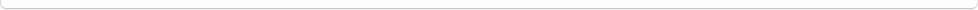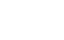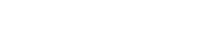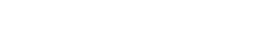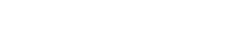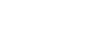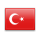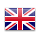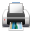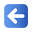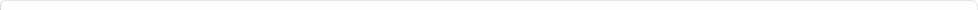Description of Individual Course Units
 Course Unit Code Course Unit Title Type of Course Unit Year of Study Semester Number of ECTS Credits 20150102002200 CALCULUS - II Compulsory 1 2 5
Level of Course Unit
First Cycle
Objectives of the Course
To give a broad knowledge and basic understanding of sequences and series and to gain ability of using the concepts of limit, continuity, partial differentiation , double integrals
Name of Lecturer(s)
Ögr. Gör. Özlem ÖZDEMİR
Learning Outcomes
 1 Student will find to convergence of sequences and series and interval of convergence of power series 2 Student will find to convergence of sequences and series and interval of convergence of power series 3 Student will use vector algebra in 3d-space and in plane and writing the equations of planes and lines 4 Student will use vector algebra in 3d-space and in plane and writing the equations of planes and lines 5 Student will understand the limit and the continuity of multivariable functions, computing partial derivatives of them, finding tangent lines, directional derivatives, gradients 6 Student will understand the limit and the continuity of multivariable functions, computing partial derivatives of them, finding tangent lines, directional derivatives, gradients 7 Student will understand solving the problem of extreme values by using second derivative test 8 Student will understand solving the problem of extreme values by using second derivative test 9 Student will evaluate double integrals and applying double integrals for computing of area and volume 10 Student will evaluate double integrals and applying double integrals for computing of area and volume
Mode of Delivery
Daytime Class
Prerequisites and co-requisities
None
Recommended Optional Programme Components
None
Course Contents
Infinite Sequences and Series : Sequences , Convergence and Divergence Limits of Sequences, The Sandwich Theorem for Sequences, The Continuous Function Theorem for Sequences, Commonly Occurring Limits, Recursive Definitions, Bounded Monotonic Sequences, Monotonic Sequences Theorem , Infinite Series, Geometric Series, The nth-Term Test for a Divergent Series, Combining Series, Adding or Deleting Terms The Integral Test , P-Series , Harmonic Series , Comparison Tests, The Comparison Test , The Limit Comparison Test , The Ratio and Roots Tests , The Ratio Test , The Root Test, Alternating Series, Absolute and Conditional Convergence , Alternating Harmonic Series , The Alternating Series Test(Leibniz’s Test), Absolute and Conditional Convergence , The absolute Convergence Test, Power Series: Power Series and Convergence, The Radius of Convergence of a Power Series , Operations on Power Series ,The Series Multiplication Theorem for Power Series , The Term-by-Term Differentiation Theorem , The Term-by Term Integration Theorem, Taylor and Maclaurin Series, Taylor Polynomial of order n , Convergence of Taylor Series , Taylor’s Theorem , Taylor’s Formula Applications of Taylor Series , Using Taylor Series , Evaluating Non elementary Integrals, Arctangents, Evaluating Indeterminate Forms, Parametric Equations and Polar Coordinates: Parametrizations of Plane Curves , Parametric Equations , Calculus With Parametric Curves , Tangents and Areas ,Length of Parametrically Defined Curve Polar Coordinates: Polar Equations and Graphs, Relating Polar and Cartesian Coordinates, Equations Relating Polar and Cartesian Coordinates, Symmetry, Symmetry Tests for Polar Graphs, Graphing in Polar, Areas and Lengths in Polar Coordinates ,Area in the Plane, Length of a Polar Curve, The Standard Polar Equation for Lines, Circles Vectors and The Geometry of Space: Three-Dimensional Coordinate Systems, Distance and Spheres in Space, Vectors, The Dot Product, Angle Between Two Vectors, Perpendicular (Orthogonal) Vectors, The Dot Product Properties and Vector Projections, The Cross Product of Two Vectors in Space, Parallel Vectors, Properties of the Cross Product, Area of a Parallelogram, The Triple Scalar Product, Lines and Planes in Space, Lines and Line Segments in Space: Vectors Equation for a Line, Parametric Equations for a Line, An Equation for a Plane in Space, Lines of Intersection, Cylinders and Quadratic Surfaces: Cylinders, Quadric Surfaces, Ellipsoids,Parabolas, Elliptical Parabolas, Elliptical Cones, Spheres, Vector-Valued Functions and Motion in Space: Curves in Space and Their Tangents, Limits and Continuity, Derivatives, Position Vector, Velocity Vector, Acceleration Vector, Differentiation Rules, Arc Length Along a Space Curve, Unit Tangent Vector, Partial Derivatives: Functions of Several Variables: Domains and Ranges , Functions of Two Variables ,Graphs and Level Curves of Functions of Two Variables,Functions of Three Variables, Limits and Continuity in Higher Dimensions, Limits for Functions of Two Variables, Continuity, Two-Path Test for Nonexistence of a Limit , Continuity of Composites, Functions of More Than Two Variables, Partial Derivatives: Functions of More Than Two Variables, Partial Derivatives and Continuity, Second-Order Partial Derivatives, The Mixed Derivative Theorem, Partial Derivatives of Still Higher Order, Differentiability, The Increment Theorem for Functions of Two Variables, The Chain Rule: Functions of Two Variables, Chain Rule for Functions of two Independent Variables, Functions of Three Variables, Chain Rule for Functions of Three Independent Variables, Functions Defined on Surfaces, Chain Rule for Two Independent Variables and Three Intermediate Variables, Implicit Differentiation Revisited, A Formula for Implicit Differentiation, Functions of Many Variables, Directional Derivatives and Gradient Vectors: Directional Derivatives in the Plane, Interpretation of the Directional Derivative, Calculation and Gradients, Gradients and Tangents to Level Curves, Functions of Three Variables, Tangent Planes and Differentials, Tangent Plane of The Surface, The Normal Line of The Surface, The Linearization of Function, Differentials, Total Differentials of Function, Extreme Values and Saddle Points: Derivative Tests for Local Extreme Values, First Derivative Test for Local Extreme Values, Critical Point, Saddle Point, Second Derivative Test for Local Extreme Values, Taylor’s Formula for functions of Two Variables at the point (a.b) , Taylor’s Formula for functions of Two Variables at the point (0.0), Partial Derivatives with Constrained Variables, Decide which Variables Are Dependent and Which Are Independent , How to Find Partial Derivative When the Variables in w=f(x,y,z) are Constrained by Another Equation, Double Integrals : Double and Iterated Integrals over Rectangles, Double Integrals, Double Integrals as Volumes, Fubini’s Theorem for Calculating Double Integrals, Fubini’s Theorem (First Form), Double Integrals over General Regions , Double Integrals over Bounded, Nonrectangular Regions, Fubini’s Theorem (Stronger Form), Finding Limits of Integration, Using Vertical Cross-sections, Using Horizontal Cross-sections, Properties of Double Integrals, Area by Double Integration, Average Value, Double Integrals in Polar Form: Integrals in Polar Coordinates, Finding Limits of Integration, Area in Polar Coordinates, Substitutions in Double Integrals, Changing Cartesian Integrals into Polar Integrals, Substitutions in Double Integrals
Weekly Detailed Course Contents
 Week Theoretical Practice Laboratory 1 Infinite Sequences and Series : Sequences , Convergence and Divergence of Sequences, The Sandwich Theorem for Sequences, The Continuous Function Theorem for Sequences, Commonly Occurring Limits, Recursive Definitions, Bounded Monotonic Sequences, Monotonic Sequences Theorem , Infinite Series, Geometric Series, The nth-Term Test for a Divergent Series, Combining Series, Adding or Deleting Terms,The Integral Test , P-Series , Harmonic Series 2 Comparison Tests, The Comparison Test , The Limit Comparison Test , The Ratio and Roots Tests , The Ratio Test , The Root Test , Alternating Series , Absolute and Conditional Convergence , Alternating Harmonic Series , The Alternating Series Test(Leibniz’s Test) , Absolute and Conditional Convergence , The absolute Convergence Test,Power Series , Power Series and Convergence,The Radius of Convergence of a Power Series 3 Operations on Power Series ,The Series Multiplication Theorem for Power Series , The Term-by-Term Differentiation Theorem , The Term-by Term Integration Theorem,Taylor and Maclaurin Series, Taylor Polynomial of order n, Convergence of Taylor Series, Taylor’s Theorem, Taylor’s Formula,Applications of Taylor Series , Using Taylor Series , Evaluating Non elementary Integrals, Arctangents, Evaluating Indeterminate Forms 4 Parametric Equations and Polar Coordinates:Parametrizations of Plane Curves , Parametric Equations , Calculus With Parametric Curves , Tangents and Areas ,Length of Parametrically Defined Curve,Polar Coordinates: Polar Equations and Graphs, Relating Polar and Cartesian Coordinates, Equations Relating Polar and Cartesian Coordinates, Symmetry, Symmetry Tests for Polar Graphs, Graphing in Polar 5 Areas and Lengths in Polar Coordinates ,Area in the Plane, Length of a Polar Curve, The Standart Polar Equation for Lines, Circles,Vectors and The Geometry of Space: Three-Dimensional Coordinate Systems, Vectors, The Dot Product, Angle Between Two Vectors, Perpendicular (Orthogonal) Vectors, The Dot Product Properties and Vector Projections, The Cross Product of Two Vectors in Space, Parallel Vectors, Properties of the Cross Product, The Triple Scalar Product 6 Lines and Line Segments in Space: Vectors Equation for a Line, Parametric Equations for a Line, An Equation for a Plane in Space, Lines of Intersection, Cylinders and Quadratic Surfaces,Vector-Valued Functions and Motion in Space:Curves in Space and Their Tangents, Limits and Continuity, Derivatives, Position Vector, Velocity Vector, Acceleration Vector, Differentiation Rules, Arc Length Along a Space Curve, Unit Tangent Vector 7 Functions of Several Variables: Domains and Ranges , Functions of Two Variables ,Graphs and Level Curves of Functions of Two Variables,Functions of Three Variables, Limits and Continuity in Higher Dimensions, Limits for Functions of Two Variables, Continuity, Two-Path Test for Nonexistence of a Limit , Continuity of Composites, Functions of More Than Two Variables,Partial Derivatives: Functions of More Than Two Variables, Partial Derivatives and Continuity 8 Mid-term exam 9 Second-Order Partial Derivatives,Partial Derivatives of Still Higher Order, Differentiability,The Chain Rule: Functions of Two Variables , Chain Rule for Functions of two Independent Variables, Functions of Three Variables, Chain Rule for Functions of Three Independent Variables, Functions Defined on Surfaces, Chain Rule for Two Independent Variables and Three Intermediate Variables 10 Implicit Differentiation Revisited, A Formula for Implicit Differentiation,Directional Derivatives and Gradient Vectors , Directional Derivatives in the Plane , Interpretation of the Directional Derivative , Calculation and Gradients , Gradients and Tangents to Level Curves , Functions of Three Variables, Tangent Planes and Differentials, Tangent Plane of The Surface, The Normal Line of The Surface, The Linearization of Function, Differentials , Total Differentials of Function 11 Extreme Values and Saddle Points: Derivative Tests for Local Extreme Values, First Derivative Test for Local Extreme Values, Critical Point, Saddle Point , Second Derivative Test for Local Extreme Values, Taylor’s Formula for functions of Two Variables at the point (a.b) , Taylor’s Formula for functions of Two Variables at the point (0.0) 12 Partial Derivatives with Constrained Variables, Decide which Variables Are Dependent and Which Are Independent , How to Find Partial Derivative When the Variables in w=f(x,y,z) are Constrained by Another Equation,Double Integrals : Double and Iterated Integrals over Rectangles, Double Integrals, Double Integrals as Volumes, Fubini’s Theorem for Calculating Double Integrals 13 Fubini’s Theorem (First Form), Double Integrals over General Regions , Double Integrals over Bounded, Nonrectangular Regions , Fubini’s Theorem (Stronger Form) , Finding Limits of Integration ,Using Vertical Cross-sections , Using Horizontal Cross-sections , Properties of Double Integrals, Area by Double Integration, Average Value 14 Double Integrals in Polar Form: Integrals in Polar CoordinatesFinding Limits of Integration, Area in Polar Coordinates, Substituons in Double Integrals, Changing Cartesian Integrals into Polar Integrals, Substitutions in Double Integrals
Recommended or Required Reading
1. Thomas’ Calculus, 12th Edition, G.B Thomas, M.D.Weir, J.Hass and F.R.Giordano, Addison-Wesley, 2012.
Planned Learning Activities and Teaching Methods
Assessment Methods and Criteria
 Term (or Year) Learning Activities Quantity Weight Midterm Examination 1 100 SUM 100 End Of Term (or Year) Learning Activities Quantity Weight Final Examination 1 100 SUM 100 Term (or Year) Learning Activities 40 End Of Term (or Year) Learning Activities 60 SUM 100
Language of Instruction
Turkish
Work Placement(s)
None